### Home > CALC > Chapter 10 > Lesson 10.1.5 > Problem10-41

10-41.

Find two different series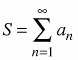and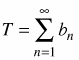(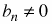) such that S and T both diverge yet the series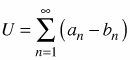converges. Homework Help ✎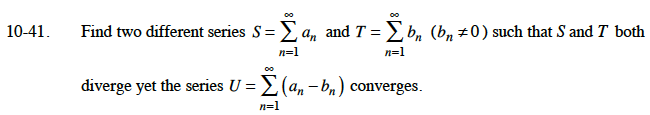Any series of the following form diverge. Use series of this form to determine your answer.

$\sum_{n=1}^{\infty}\frac{a}{bn+c}$